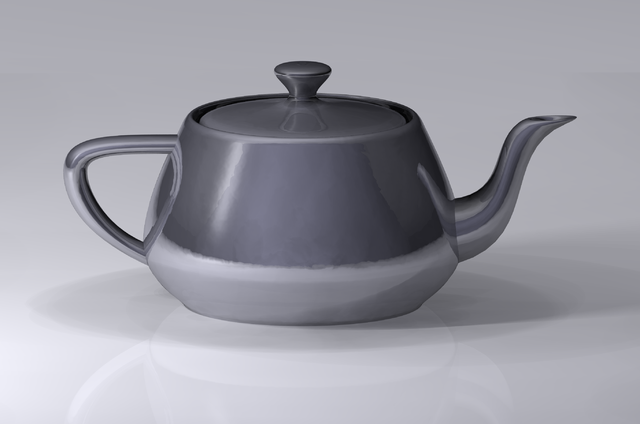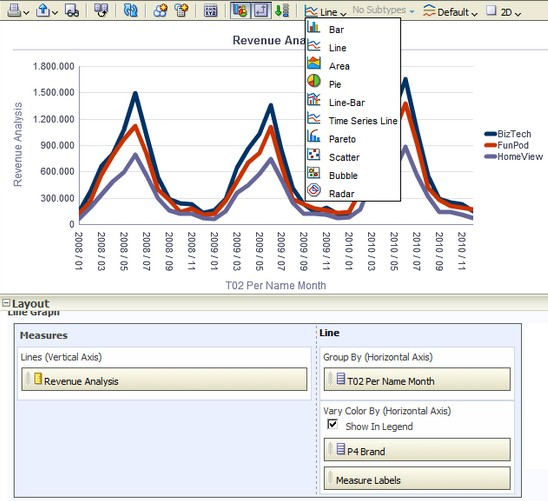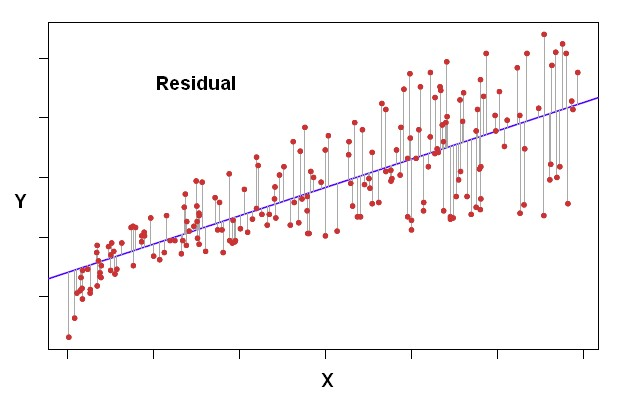# Data Visualisation - Scatterplot

A Scatterplot displays x-y values as discrete points, scattered within an x-y grid.

It plots data points based on two independent variables.

Scatter graphs are useful for observing pattern in large data sets:

## Matrix (SPLOM)

A scatter plot matrix is a very nice way of plotting every variable against every other variable.

They are used to investigate the relationship between the variables.

## Documentation / Reference

Discover MoreData Visualization - Visual (or Mark)

A visual is an object created from visual primitive that gets the value of its properties from the data. See also: Visual Encoding Bar (ie rectangle) Point (ie Circle or Square) Line Area...Data Visualization Foundation

The Utah Teapot. Data visualization is the process of mapping quantitative data to visuals (shapes, color, position, etc) to create a graph made of geometric object. Information visualization: ...Data Viz - Raster Plot

A Scatter plot is not practical when visualizing millions or billions of dots representing the intersections of the two variables. A Raster plot takes two continuous variables and creates discrete 2-dimensional...Ggplot - Point (geom_point, geom_jitter) (Scatterplot)

A geom point to create Scatterplot geom_jitter is a shortcut for geom_point(position = “jitter”) Points are jittered to reduce overplotting.OBIEE 10G/11g - (Chart|Graph) View

A graph view displays numeric information visually, which makes it easier to understand large quantities of data. For displaying a single data value, a gauge is often more effective than a graph. Graphs...Plotly - Line and Scatterplot (Plot|Chart)

Line and ScatterplotR - Scatterplot

Useful way to see all plots for a two-class variable Standard scatterplot Standard scatterplot with regression line Scatterplot matrix Color scatterplot matrix, colored and ordered by...Statistics - (Residual|Error Term|Prediction error|Deviation) (e| )

The residual is a deviation score measure of prediction error in case of regression. The difference between an observed target and a predicted target in a regression analysis is known as the residual...Viz - Seven Basic Tools of Quality

The Seven Basic Tools of Quality is a designation given to a fixed set of graphical techniques identified as being most helpful in troubleshooting issues related to quality. The seven tools are: ...